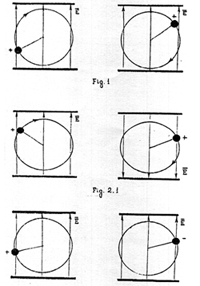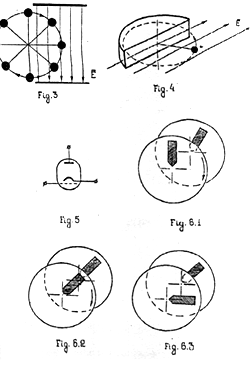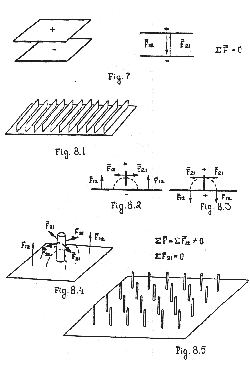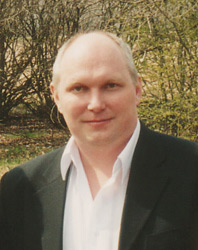The Application of Potential Energy for Creation of Power
By Alexander V. Frolov

Professional physicist's dislike for questions about applications of potential energy for power creation is understandable since they have a strong orientation to the Law of conservation of Energy.

This paper, without formulas demonstrates that there are no prohibitions to free energy in Nature.
The availability of the potential field is a sufficient condition for creation of fuelless power systems.
When such a system is in operation then certain effects show up that are connected with the Law of
Conservation of Energy It is necessary now to formulate this Law in a general way.

The problem of without-support (reactionless) motion may be solved by means of asymmetrical potential interaction which is also proposed.

1. The Total Work of a Potential Field

There are "incontestable" formulations: the work of potential field along closed trajectory is equal to zero. Indeed, from Figure 1 we can see that part of the work is positive (acceleration) and another part is negative (deceleration).In physics textbooks the task of application of potential for making only positive work is not treated. This reluctance can be explained by unwillingness of textbooks authors to discuss more interesting question of energy transformations when the inner structure of space-time is changed.

Fig. 2 shows the same charged mass M rotating in potential electric field. The difference is in mode of interaction. The polarity of the interaction is changed when positive work is changed to negative.
Figure 2.1 shows that the direction of the field is changed and Fig. 2.2 shows that the sign of the
charge is changed. There are simple examples of the application of potential field for creation of only positive work in a load. Fig. 3 depicts a scheme that is similar to water mill wheel.Now let's return to Fig. 1. Have you heard of the miller who submerged the mill wheel in the water flow of the river and then "scientifically" proved that it is impossible to use wafer flow for creation of power?  Unfortunately, our school teachers use similar arguments!

So, part of the trajectory or part of the periodical cycle that is part of negative work (deceleration) can be excluded by means of spatial superposition, pulsed mode operation, change of polarity of
interaction, or by means of suitable screening (see Fig. 4). Fig. 5 shows that some changes in
arrangement of electrodes in a vacuum tube allows one to increase energy of emission of the flow of
electrons. These schemes require certain development for industry application, of course. Note that Coulomb's interaction is inversely proportional to square of distance so it is necessary to try to decrease this  distance. Fig. 6 shows a version of a free energy device similar to a well-known electric machine.  Let's consider the interaction of two segments which have opposite charges, for example. In case of constant sign of interaction the process corresponds to the conclusions of the above miller (who immersed his mill wheel). Fig. 6.1 shows that attraction between segments create propulsion force and rotor moves with some acceleration, then Fig. 6.2 is at the point of balance and Fig. 6.3 shows that the rotor is decelerated since segments remain in attraction.

The total work of potential field is equal to zero. But why? It is not an indispensable condition of Nature. It is enough to change the sign of interaction between segments after balance point from
attraction to repulsion to create the mode of acceleration again!

Let's omit technological details and description of contacts, collector brushes, condenser, transformers and so on.

Above we considered examples of scalar electric potential application. If is possible to use any
potential for it, for example vector potential of magnetic field and also the gravitational potential.

It is not new that a potential field can create work. Any textbook shows examples of  negative-positive cycle of such work and proves that total work of successive acceleration and deceleration is equal to zero. But since it is successive performance of two processes it is conceptually possible to change parameters of one process to create cycle as sum of
two or several parts of positive work. The solution lies in point-momentum of balance.

2. The Asymmetrical Potential Interaction.

Let's consider the application of potential field for creation of propulsion force and without-support (reactionless) motion. Fig. 7 is a scheme using ordinary flat electric condenser.Two flat plates are in attraction (different charges)  or in repulsion  (same charges). Total force equals zero and the material system of the condenser remains stationary. All we need to find a solution is to note that the force line of potential field always is perpendicular to the charged surface. Fig. 8 shows the same condenser but one plate is perpendicularly turned to another plate.

The vertical plate is divided into several elements to decrease the distance of Coulomb's interaction between plates. Total force that influence any vertical element is the sum of opposite forces and it is equal to zero. But total force that is applied to another (horizontal) plate is sum of co-directional elementary forces and it is not equal to zero. The system can be caused to move by means of asymmetrical potential interaction.
Note that perpendicular elements can have a cylindrical shape. The propulsion force can be regulated by means of changing the angle between plates of an asymmetrical condenser or by means of changing potential.

The well-known experiments with "inertioidal" moving systems can be considered as a particular
case of asymmetrical (vector) potential technology. When eccentric mass is moving along epicycloidal or cardioidal heart-shaped trajectory, the trajectory creates a propulsion force that is the result of the gradient of acceleration. This process has been described by using a vector potential concept. Since asymmetrical scalar potential interactions do not require additional power after its creation, the scalar technology is more interesting for spacecraft applications than inertioidal systems.

3. The Effects of Space-Time Disbalance

Now we have a common sense question: Where do we extract power from if we use potential energy to create work? I must note that the existence of force effects of potential fields must raise the same question. For example, why can a permanent magnet (the vector potential) hold a massive piece of metal when it makes work against the gravity force and the magnet doesn't require power?
The Whittaker's conception of potential as bidirectional flow of energy is discussed by T.E.
Bearden  . The generalization of Newton's Third Law in this conception leads to a requirement for a complementary pair for the process of electromagnetic radiation. Since the process means the change of information (of structure of space) in this pair anti-process takes place in reverse-
time. Certainly, anti-process is developed from own past time to future hut from our point of view if is changed from future to past time. By generalized Third Law of Newton, the inner structure of electric potential field is created by two oncoming flows of energy: the photons are spreading from the charge-source of the potential field and the anti-photons are spreading  into the source of the charged mass. So, there is the question: Since "something" cannot spring from "nothing" what and where is something "reduced" (changed) if in local region of our space-time the free energy process occurs and some power is provided to the load?
Let's make a generalization of this question. By reading T.E. Bearden we find that "potential" and "energy" is the same. Then considering "energy" as "ordering and disordering" of the spatial structure we conclude that energy is the description of any process. Considering that a "process" is a change of information or spatial structure in time from the point of view of the observer explains the effects that are connected with changes of natural potential field energy balance.

Therefore, the potential field can be considered as a bidirectional process/anti-process. Usually
energy and information that is radiated by the source of the potential field (the direct process in time) is somewhat different from energy and information that is received (the anti-process in reversetime). This difference determine the rate of time for this mass object and the existence of this mass as the source of the potential field. This difference is equal to trapped energy of mass-form that can be
transformed as radiation energy in case of mass decay.

If the potential field is used only for the creation of positive work in conformance with the Law of
Conservation of Energy the power process in space-time must be balanced by corresponding
power anti-process in space of reverse-time and this effect shows itself as a local change of spatial
curvature and rate of flow of time.

If it is necessary for design of practical  applications  it is possible to calculate the amount of
power that must be dispersed as heat to obtain the mode of total compensation of natural spatial planet curvature and rate of time in local area of space-time.

So it is impossible to create "something" from "nothing" but if the creation of some power process
is balanced by corresponding anti-process you don't create "something" in a free energy system from the point of view of Eternity. After Whittaker's representation of the electron as a function of two
scalar potentials the old philosophical idea of the unreality of material existence has the possibility of
experimental verification. The departure from static description of mass to a dynamic description of the material world allows one to develop an improved conception of reality. Since the mass (and matter) is a process that is balanced by reverse-process there are no difficulties of principle to develop such technologies as transmutation of chemical elements , materialization and dematerialization, teleportation and so on.

The application of potential energy for the creation of power does not violate the Law of Conservation of Energy that remain true in a more general view: The complete energy of a four-dimensional system is the sum of the energy of space-time processes and the energy of space-reverse-time processes. and it is constant and equal in sum to zero.

References